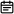# 771. 宝石与石头2019-06-18

## 题目链接

https://leetcode-cn.com/problems/jewels-and-stones/

## 题目描述

`J` 中的字母不重复，`J` 和 `S` 中的所有字符都是字母。字母区分大小写，因此`"a"``"A"`是不同类型的石头。

``````输入: J = "aA", S = "aAAbbbb"

``````输入: J = "z", S = "ZZ"

• S 和 J 最多含有50个字母。
• J 中的字符不重复。

## 解题方案

### 思路

• 标签：字符串
• 首先对J进行遍历，将字符分别存到HashSet中，以便之后遍历S的时候查找
• 遍历S，并将每个字符与HashSet中的进行比对，如果存在，则结果ans++，遍历结束，返回ans
• 时间复杂度：O(m+n)，m为J的长度，n为S的长度

### 代码

• Java版本
``````class Solution {
public int numJewelsInStones(String J, String S) {
Set<Character> set = new HashSet<>();
for(int i = 0; i < J.length(); i++) {
}
int ans = 0;
for(int i = 0; i < S.length(); i++) {
if(set.contains(S.charAt(i))){
ans++;
}
}
return ans;
}
}``````
• JavaScript版本
``````/**
* @param {string} J
* @param {string} S
* @return {number}
*/
var numJewelsInStones = function(J, S) {
const set = new Set();
for(const s of J) {
}
let ans = 0;
for(const s of S) {
if(set.has(s)){
ans++;
}
}
return ans;
};``````

### 画解